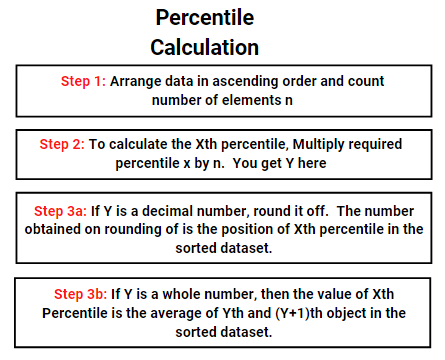# Calculating Percentiles, Quartiles, and Quintiles

Statistics is a branch of Mathematics that does a huge analysis to create information from data using some formula and representation techniques.

Percentiles, Quartiles, and Quintiles are some of the tools used to extract information, let us see what they are in upcoming sections.

## Percentiles

• Percentile is a kind of measure used to express data below a specific per cent. E.g.: Ram got 25 Percentile in the CAT exam; this means that 25% of the total students who appeared in the CAT exam are below Ram.
• Like a coin that has two faces, percentile has two interpretations e.g. Ram got 25 Percentile in CAT exam; this means that 75% of the total students who appeared in the CAT exam are above Ram.
• Percentile speaks nothing about the absolute value rather it speaks about the position of an element in overall data set e.g. Ram got 25 Percentile in CAT exams, this does not tell about the scores that Ram got, it rather tells us about the position of Ram in overall exams.
• Percentile is used where the position of an element is of matter and not its value.

### Percentile Calculation

#### Example 1:

• Let us take a data set = 1, 3,5,7,9,2,4,6,8,10,12,13,11,20 and find 20th and 50th percentile in it.
• Solution:
• Step 1: Arrange data in ascending order and count number of elements: 1,2,3,4,5,6,7,8,9,10,11,12,13,20 (total elements n = 14)
• Step 2: Multiply required percentile by n i.e. 0.20 * 14 = 2.80 and 0.50 * 14 = 7.00
• Step 3a: If the result of Step 2 is a decimal number round it off, count this number from left to right in sorted data set, this is the answer i.e. 2.80 becomes 3.00 on rounding, 3rd number from left is 3, hence 20th percentile of above data is 3
• Step 3b: If the result of Step 2 is the whole number, then the answer is the average of the value of this number and its corresponding number in data set i.e. 50th percentile is an average of the value of 7th and 8th position in data set. Hence, 50th percentile = (7+8)/2 =7.5

#### All the steps followed above in example 1, can be written as:## Quartiles

• It is the same as a percentile, quartiles as the name suggest a deal with quarters. It divides data into 4 equal quarters or parts
• Hence, in any data there can be 4 Quartiles viz. 25 Percentile, 50 Percentile, 75 Percentile, and 100 Percentile.
• 25 Percentile known as Lower Quartile (Q1), 50 Percentile is known as Middle Quartile (Q2) and the 75 Percentile is known as Upper Quartile (Q3), the last one is the 100th Percentile itself.
• Another term is Interquartile Range = Q3 – Q1.
• Quartile can be calculated by the method of calculating Percentile as shown above by calculating values of 25, 50, 75 Percentile.

## Quintiles

• It is the same as a percentile, unlike Quartile, Quintiles divide the complete data set in 5 parts. Hence, in any data, there are 5 Quintiles which divide the complete data set in 5 equal parts.
• The Quintile points are 20 percentile, 40 percentile, 60 percentile, 80 percentile, and 100 percentile. The five quintiles are known as the first quintile, second quintile, third quintile, fourth quintile, and fifth quintile.
• Quintiles can easily be calculated by the method of calculating Percentile as shown above.

### Example of Quartile and Quintile

#### Example 2:

• Let us take a data set = 1, 3,5,7,9,2,4,6,8 (N =9) and find all percentile and Quantiles.
• Solution:
• Sorting it in Ascending Order: 1,2,3,4,5,6,7,8,9
• First Quartile = 25th Percentile = 2nd Element = 2
• Second Quartile = 50th Percentile = 5th Element = 5
• Third Quartile = 75th Percentile = 7th Element =7
Similarly,
• First Quintile = 20th Percentile = 2nd Element = 2
• Second Quintile = 40th Percentile = 4th Element = 4
• Third Quintile = 60th Percentile = 5th Element = 5
• Fourth Quintile = 80th Percentile = 7th Element =7